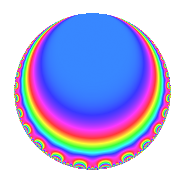# Properties

 Label 1800.1.l.aLevel 1800 Weight 1 Character orbit 1800.l Analytic conductor 0.898 Analytic rank 0 Dimension 2 Projective image $$S_{4}$$ CM/RM No Inner twists 2

# Related objects

## Newspace parameters

 Level: $$N$$ = $$1800 = 2^{3} \cdot 3^{2} \cdot 5^{2}$$ Weight: $$k$$ = $$1$$ Character orbit: $$[\chi]$$ = 1800.l (of order $$2$$ and degree $$1$$)

## Newform invariants

 Self dual: No Analytic conductor: $$0.898317022739$$ Analytic rank: $$0$$ Dimension: $$2$$ Coefficient field: $$\Q(\sqrt{-2})$$ Coefficient ring: $$\Z[a_1, \ldots, a_{11}]$$ Coefficient ring index: $$1$$ Projective image $$S_{4}$$ Projective field Galois closure of 4.2.10800.2 Artin image size $$48$$ Artin image $\GL(2,3)$ Artin field Galois closure of 8.2.1399680000.1

## $q$-expansion

Coefficients of the $$q$$-expansion are expressed in terms of $$\beta = \sqrt{-2}$$. We also show the integral $$q$$-expansion of the trace form.

 $$f(q)$$ $$=$$ $$q - q^{7} +O(q^{10})$$ $$q - q^{7} -\beta q^{11} + q^{13} + \beta q^{17} + q^{19} -\beta q^{23} -\beta q^{29} + q^{31} + q^{43} -\beta q^{47} + \beta q^{59} + q^{61} - q^{67} + \beta q^{77} + \beta q^{83} - q^{91} + q^{97} +O(q^{100})$$ $$\operatorname{Tr}(f)(q)$$ $$=$$ $$2q - 2q^{7} + O(q^{10})$$ $$2q - 2q^{7} + 2q^{13} + 2q^{19} + 2q^{31} + 2q^{43} + 2q^{61} - 2q^{67} - 2q^{91} + 2q^{97} + O(q^{100})$$

## Character Values

We give the values of $$\chi$$ on generators for $$\left(\mathbb{Z}/1800\mathbb{Z}\right)^\times$$.

 $$n$$ $$577$$ $$901$$ $$1001$$ $$1351$$ $$\chi(n)$$ $$1$$ $$1$$ $$-1$$ $$1$$

## Embeddings

For each embedding $$\iota_m$$ of the coefficient field, the values $$\iota_m(a_n)$$ are shown below.

For more information on an embedded modular form you can click on its label.

Label $$\iota_m(\nu)$$ $$a_{2}$$ $$a_{3}$$ $$a_{4}$$ $$a_{5}$$ $$a_{6}$$ $$a_{7}$$ $$a_{8}$$ $$a_{9}$$ $$a_{10}$$
1601.1
 1.41421i − 1.41421i
0 0 0 0 0 −1.00000 0 0 0
1601.2 0 0 0 0 0 −1.00000 0 0 0
 $$n$$: e.g. 2-40 or 990-1000 Significant digits: Format: Complex embeddings Normalized embeddings Satake parameters Satake angles

## Inner twists

Char. orbit Parity Mult. Self Twist Proved
1.a Even 1 trivial yes
3.b Odd 1 yes

## Hecke kernels

This newform can be constructed as the kernel of the linear operator $$T_{7} + 1$$ acting on $$S_{1}^{\mathrm{new}}(1800, [\chi])$$.